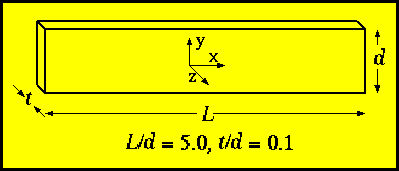# µMAG Standard Problem #2Problem suggested by Tom Koehler, IBM Almaden, ret., and proposed by H. Neal Bertram, Alfred Liu, and Chris Seberino, Center for Magnetic Recording Research, University of California at San Diego.

Please send comments to rmcmichael@nist.gov and join the µMAG discussion e-mail list for ongoing discussion.

A set of solutions have been submitted.

## Specifications

This standard micromagnetic problem includes both magnetostatic and exchange energies, but has the advantage of only one scaled parameter. If crystalline anisotropy is neglected and the geometry is fixed, scaling of the static micromagnetic equations (Brown's equations) yield a hysteresis loop which depends only on the scaled geometry to the exchange length when expressed as M/Ms versus H/Hm, where Hm = Ms (SI) or 4piMs (cgs emu). The exchange length is lex = (A/Km)1/2, where A is the exchange stiffness constant and Km is a magnetostatic energy density, Km = 1/2µ0Ms2 (SI) or 2piMs2 (cgs emu).

The field should be applied in the [1,1,1] direction, approximately 54.74 degrees ( arccos[1/root(3)] ) from each of the coordinate axes. This field orientation is chosen to avoid potential symmetry breaking problems.

#### Geometry:

Let us take a thin film of thickness t, width d, and length L. We suggest to make the problem virtually 2D by choosing t/d = 0.1, and to obtain interesting non-uniform reversal modes, L/d = 5.

#### Material parameters:

• The magnetostatic exchange length, lex
• Zero magnetocrystalline anisotropy

#### Desired output for comparison:

Calculated as a function of d/lex, with aspect ratios held constant at t/d = 0.1 and L/d = 5.0,
• Coercivity (Hc/Hm, the magnitude of the field at which the projection of the magnetization along the field, Mx+ My+ Mz, is zero.)
• Remanence ( Mx/Ms, My/Ms, Mz/Ms, at H = 0)
Please see the µMAG standard problem strategy page for information on publicizing your results.